TitleIntermediate Algebra
Answer/Discussion to Practice Problems
Tutorial 8: Introduction to Problem SolvingAnswer/Discussion to 1a The sum of a number and 2 is 6 less than twice that number.

 Make sure that you read the question carefully several times.  Since we are looking for a number, we will let  x = a number

 Step 2:  Devise a plan (translate).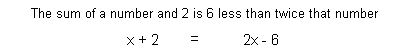Step 3:  Carry out the plan (solve).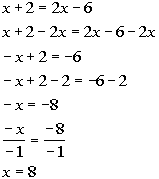*Get all the x terms on one side *Inv. of add 2 is sub. 2   *Inv. of mult. by -1 is div. by -1

 If you take the sum of 8 and 2 it is 6 less than twice 8, so this does check.   FINAL ANSWER:  The number is 8. (return to problem 1a)Answer/Discussion to 1b Find 72% of 35.

 Make sure that you read the question carefully several times.  Since we are looking for a number, we will let  x = the number

 Step 2:  Devise a plan (translate).Step 3:  Carry out the plan (solve).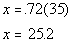*Multiply

 25.2 is 72% of 35.   FINAL ANSWER:  The number is 25.2. (return to problem 1b)Answer/Discussion to 1c A local furniture store is having a terrific sale.  They are marking down every price 45%.  If the couch you have our eye on is \$440 after the markdown, what was the original price?   How much would you save if you bought it at this sale?

 Make sure that you read the question carefully several times.  Since we are looking for the price of the couch before the markdown, we will let  x = the price of the couch

 Step 2:  Devise a plan (translate).Step 3:  Carry out the plan (solve).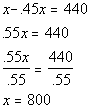*Combine like terms (1 - .45 = .55) *Inv. of mult. by .55 is div. by .55

 If you subtract the 45% markdown form 800 you do get 440.   FINAL ANSWER:  The original price is \$800. You save 800 - 440 = \$360. (return to problem 1c)Answer/Discussion to 1d A rectangular garden has a width that is 8 feet less than twice the length.  Find the dimensions if the perimeter is 20 feet.

 Make sure that you read the question carefully several times.  We are looking for the length and width of the rectangle.  Since width can be written in terms of length, we will let L = length Width is 8 feet less than twice the length: 2L - 8 = width

 Step 2:  Devise a plan (translate).Step 3:  Carry out the plan (solve).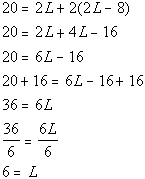*Mult. (  ) by 2 *Combine like terms *Inv. of sub. 16 is add 16 *Inv. of mult. by 6 is div. by 6

 If length is 6, then width, which is 8 feet less than twice the length, would have to be 4.  The perimeter of a rectangle with width of 4 feet and length of 6 feet is 20 feet. FINAL ANSWER:  Width is 4 feet. Length is 6 feet. (return to problem 1d)Answer/Discussion to 1e Complimentary angles, sum up to be 90 degrees.  Find the measure of each angle in the figure below.  Note that since the angles make up a right angle, they are complementary to each other.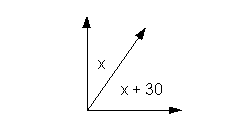Make sure that you read the question carefully several times.  We are already given in the figure that x = one angle x + 30 = other angle

 Step 2:  Devise a plan (translate).Step 3:  Carry out the plan (solve).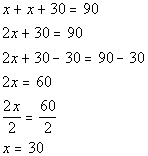*Combine like terms *Inv. of add 30 is sub. 30 *Inv. of mult. by 2 is div. by 2

 If x is 30, then x + 30 = 60.  60 and 30 do add up to be 90, so they are complementary angles. FINAL ANSWER: The two angles are 30 degrees and 60 degrees. (return to problem 1e)Answer/Discussion to 1f The sum of 3 consecutive odd integers is 57.  Find the integers.

 Make sure that you read the question carefully several times.  We are looking for 3 ODD consecutive integers, we will let x = 1st consecutive odd integer x + 2 = 2nd consecutive odd integer x + 4  = 3rd  consecutive odd integer

 Step 2:  Devise a plan (translate).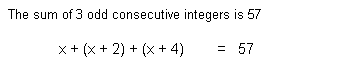Step 3:  Carry out the plan (solve).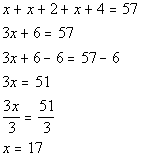*Combine like terms *Inv. of add 6 is sub. 6 *Inv. of mult. by 3 is div. by 3

 If you take the sum of 17, 19 and 21 you do get    FINAL ANSWER:  The three consecutive odd integers are 17, 19 and 21. (return to problem 1f)Answer/Discussion to 1g The cost C to produce x numbers of VCR's is C = 1000 + 100x.  The VCR's are sold wholesale for \$150 each, so the revenue is given by R = 150x.

 Make sure that you read the question carefully several times.  We are looking for the number of VCR's needed to be sold to break even, we will let x = the number of VCR's

 Step 2:  Devise a plan (translate).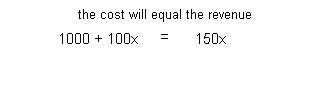Step 3:  Carry out the plan (solve).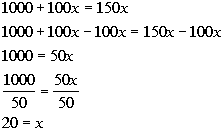*Get all the x terms on one side   *Inv. of mult. by 50 is div. by 50

 When x is 20 the cost and the revenue both equal 3000.   FINAL ANSWER: 20 VCR's. (return to problem 1g)

Last revised on July 1, 2011 by Kim Seward.
All contents copyright (C) 2002 - 2011, WTAMU and Kim Seward. All rights reserved.